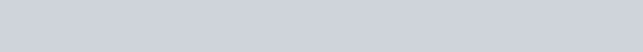Find the number of different words
Question:

Find the number of different words that can be formed from the letters of the word ‘TRIANGLE’ so that no vowels are together.

Solution:

We know that,

nPr

$=\frac{n !}{(n-r) !}$

According to the question,

Total number of vowels letter =3,

Total number of consonants letter =5The vowels can be placed in

6P3 = 6!/3! = 120

The number of way consonants can be arranged placed =5! =120

Therefore, total number of ways it can be arranged =5!×6P3=120×120 =14400